# python笔记

## 03x10 day1

``````a = 1
print(type(a))``````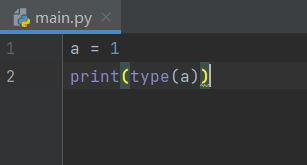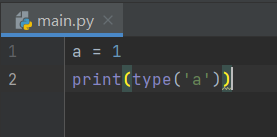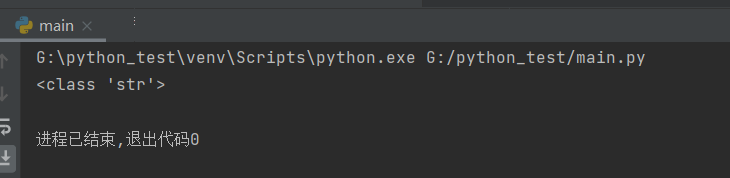``````a = 10
print('a的值是%d' %a)``````

c语言: `printf("a的值是%d",&a);`

python:`print('a的值是%d' %a)`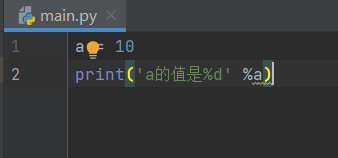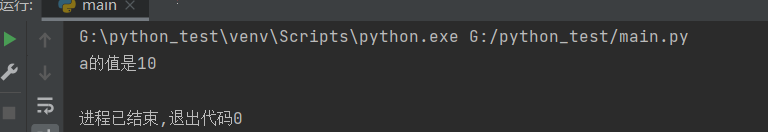``print('name: %s age: %d' % ('Tom',12))``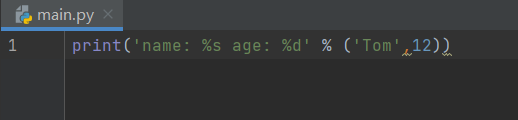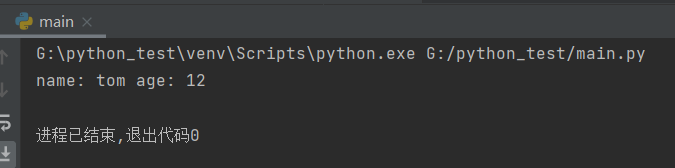### 占位符表

%c 字符
%s 字符串
%d 有符号十进制整数
%u 无符号十进制整数
%o 八进制整数
%x 十六进制整数（小写字母0x）
%X 十六进制整数（大写字母0X）
%f 浮点数
%e 科学计数法（小写’e’）
%E 科学计数法（大写’E’）
%g %f和%e的简写
%G %f和%E的简写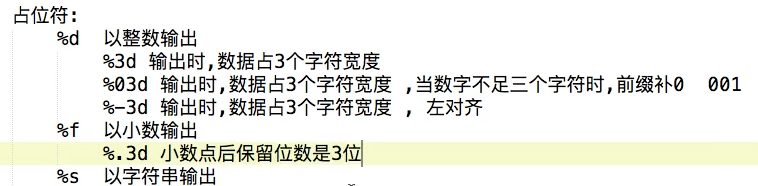### f-string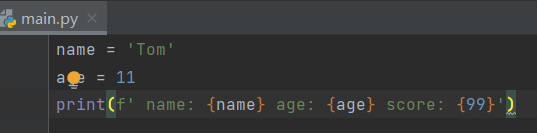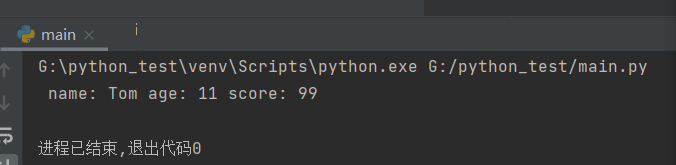`f' '`并不是必须写在print里面的 只是使用print输出 `跟%d不同`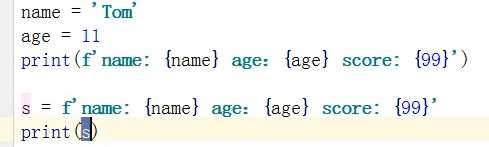### 输入函数input

``````a = input('please input a number:')
print(a)``````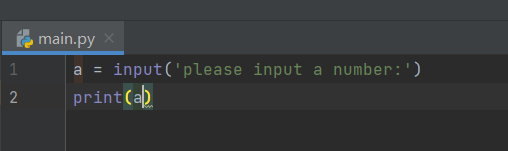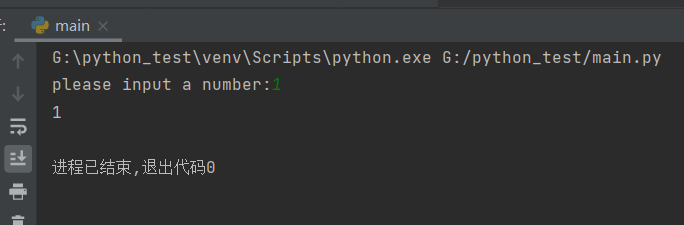input从键盘获取的数据。无论输入的是什么，得到的都是字符串类型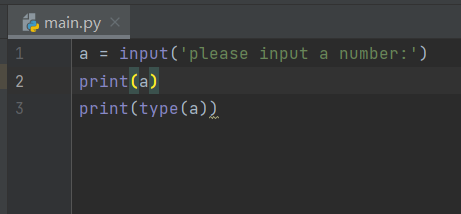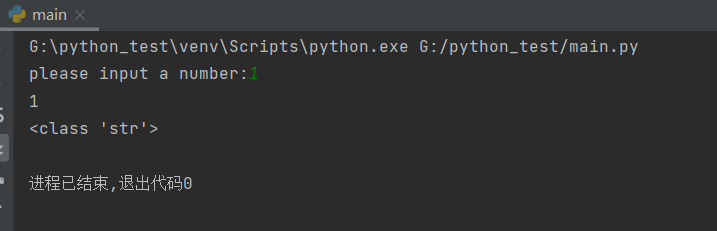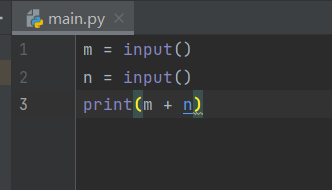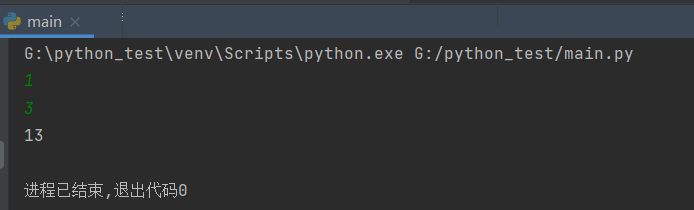### 运算符

+ 两个对象相加a + b 输出结果30
- 得到负数或是一个数减去另一个数 a - b输出结果-10
* 两个数相乘或是返回一个重复若干次的字符串a*b输出结果200
/ b/a输出结果2
// 取整除 返回商的整数部分9//2输出结果4，9.0//2.0输出结果4.0
% 取余 返回除法的余数b % a 输出结果被 0
** 指数 a**b为10的20次方，输出结果10000000000000000000

`**`高于`*` `/` `//` `%`高于 `+` `-` 不同类型的数字在进行混合运算时，整数将会转换成浮点数进行运算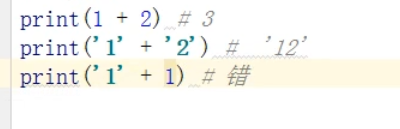### 复合赋值运算符

+= 加法赋值运算 c + = a等于c = c + a
-= 减法赋值运算 c - = a等于c = c - a
*= 乘法赋值运算 c * = a 等于c = c * a
/= 除法赋值运算 c / = a 等于 c = c / a
%= 取模赋值运算 c % = a等于c = c % a
**= 幂赋值运算 c * * = a等于c = c * * a
//= 取整除赋值运算 c / / = a等于c = c / / a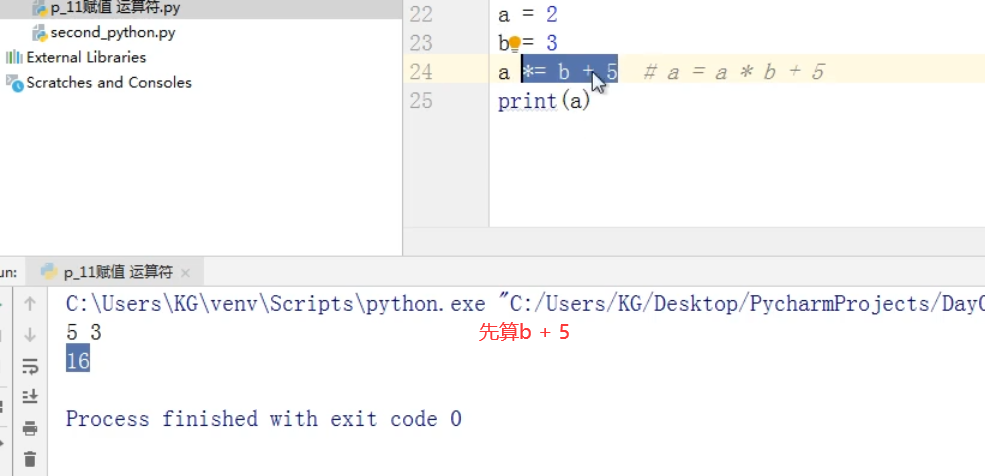### 类型转换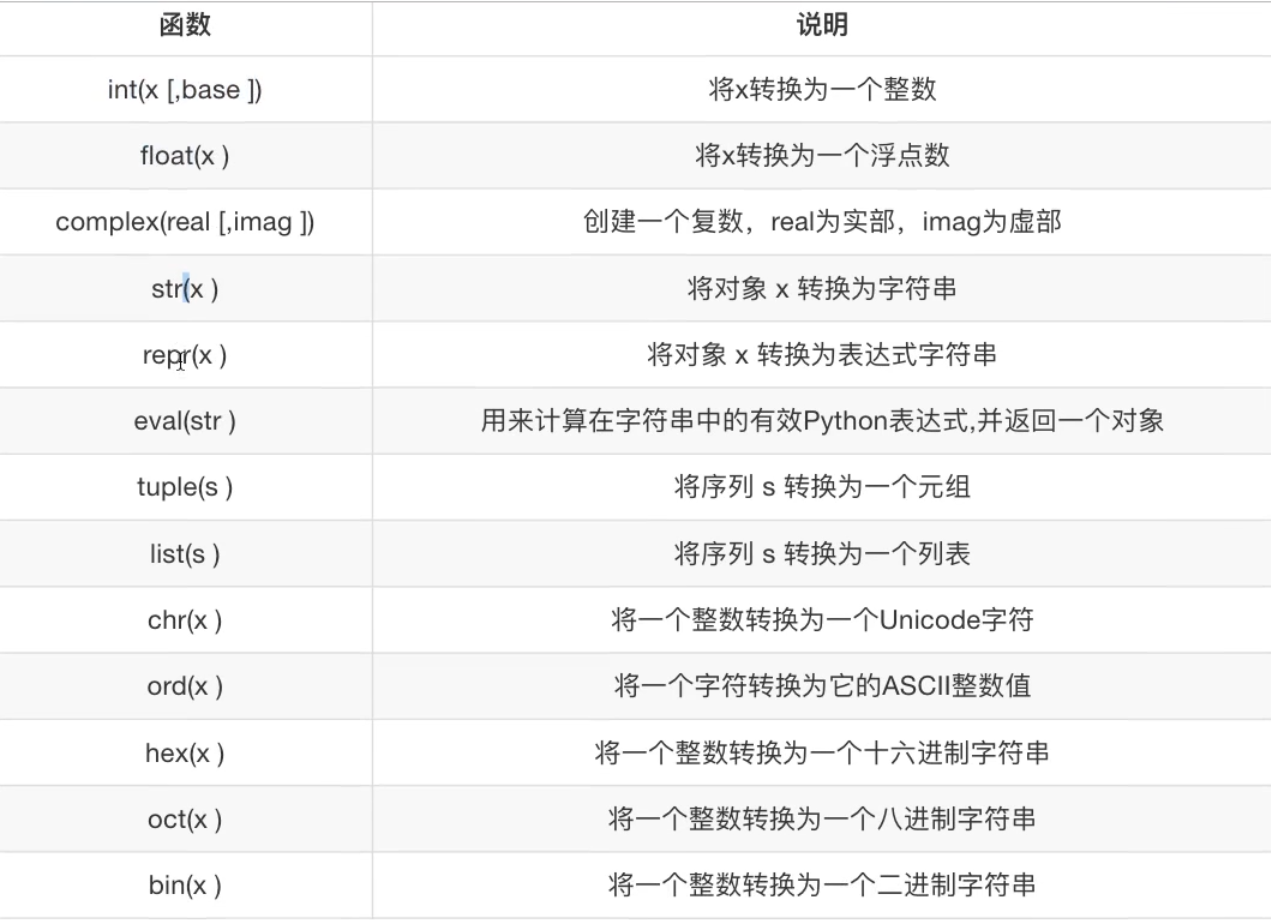print(chr(65))

print(ord(‘A’))

## 03x12 day3

### 1.函数概述

​ 在使用时，只需要使用代码的名字即可

​ 提高代码的开发效率

​ 使代码更加简洁

​ 提高代码的复用率

### 2.函数的定义和调用

``````def 函数名(参数列表...):
函数功能代码...``````
``注意：当定义完函数名回车后 写第二行代码要按tab键对齐``

``函数名（值列表...)``
``````def a():
print(123)``````

`注意：定义函数并不会执行 当后面调用时才会执行 如下图`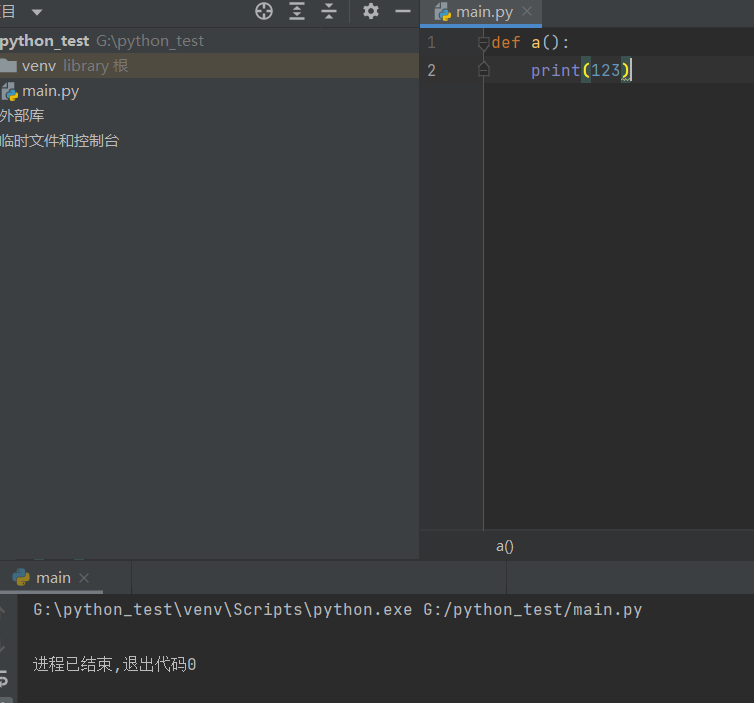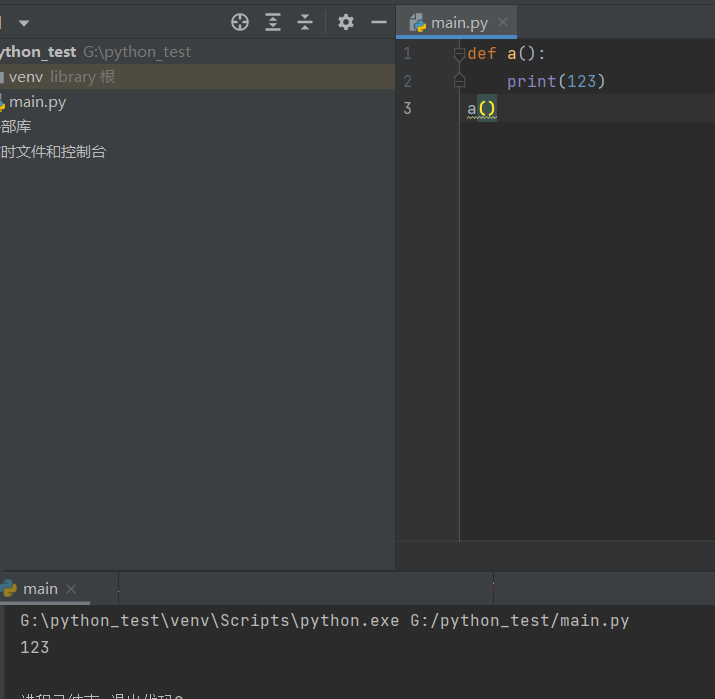### 3.函数调用过程

1.函数在定义时，函数体中的代码是不执行的

2.当产生函数调用时，程序才会跳转到函数体中执行函数的代码

3.函数体中的代码执行完成后，回到调用处

``````def show():
print('2')
print('1')
show()
print('3')``````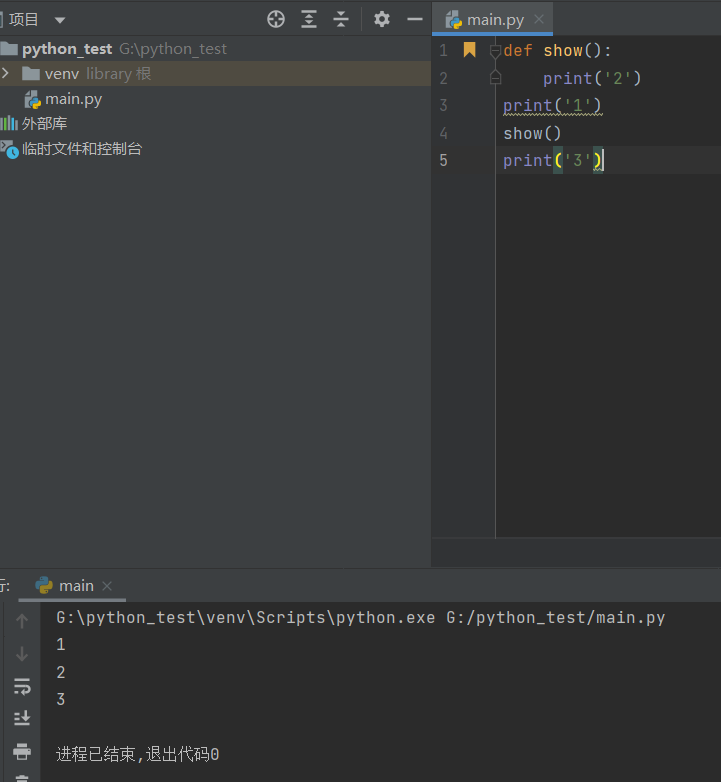### 4.函数文档说明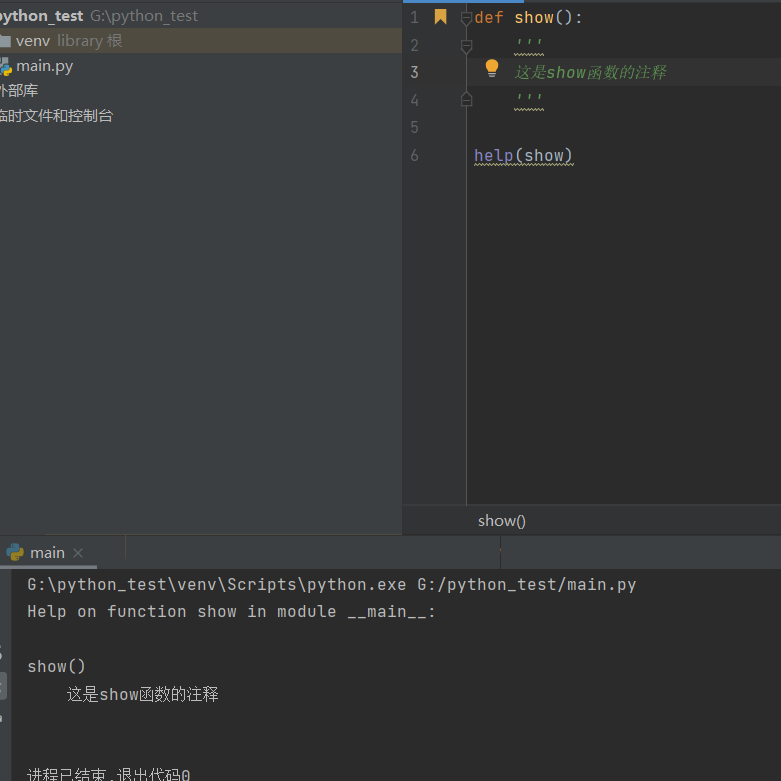### 5.函数的实参和形参

``````def say_hi(name):   #形参
print('hello', name)

say_hi('tom')       #实参``````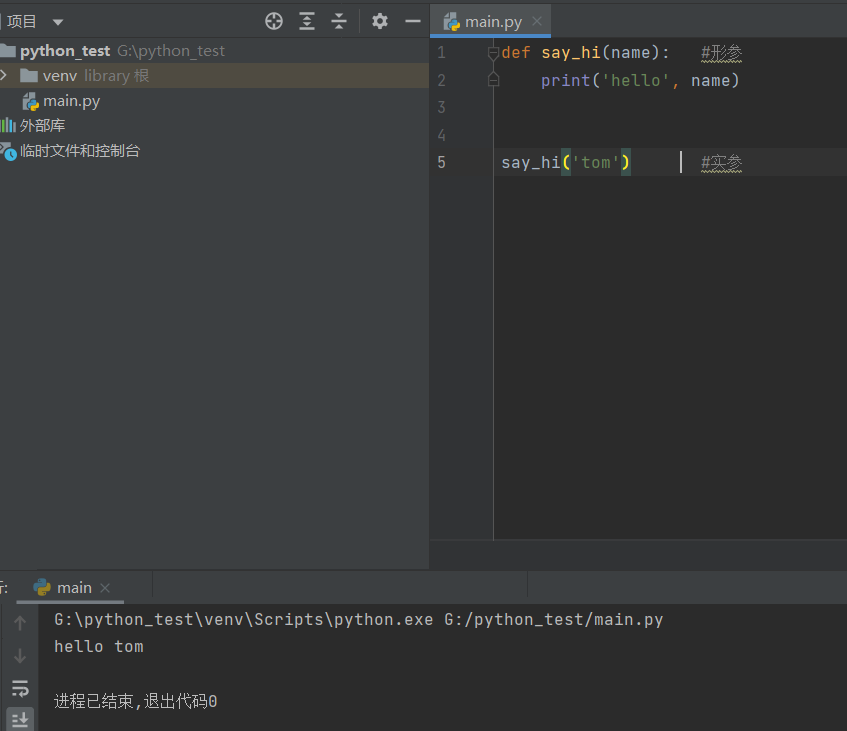### 7.函数返回值

`return` 返回一个函数的结果

`return 数据`

`return` 后面可以没有数据 返回`None`

``````def get_num():
return 1

get_num()           #不会打印输出 因为没有打印函数
print(get_num())    #会打印输出
a = get_num()       #将get_num()的值赋予给a
print(a)            #将a打印输出
``````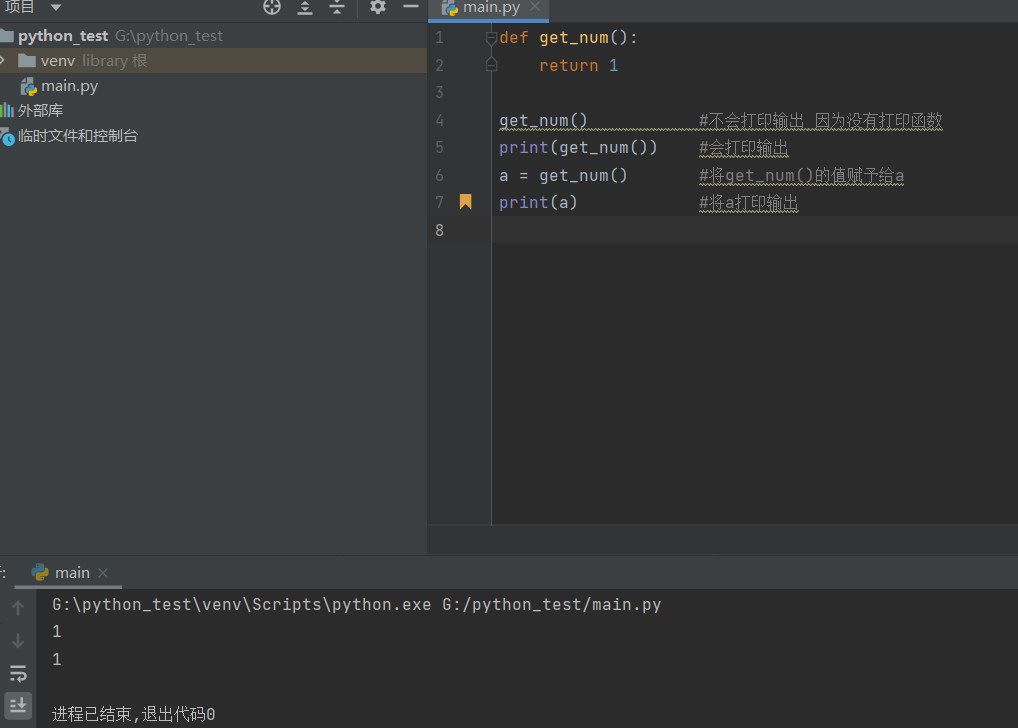``````def show():
print('1')      #输出
print('2')      #输出
return()        #停止返回至调用函数
print('3')      #不执行
print('4')      #不执行
show()              #调用show()函数``````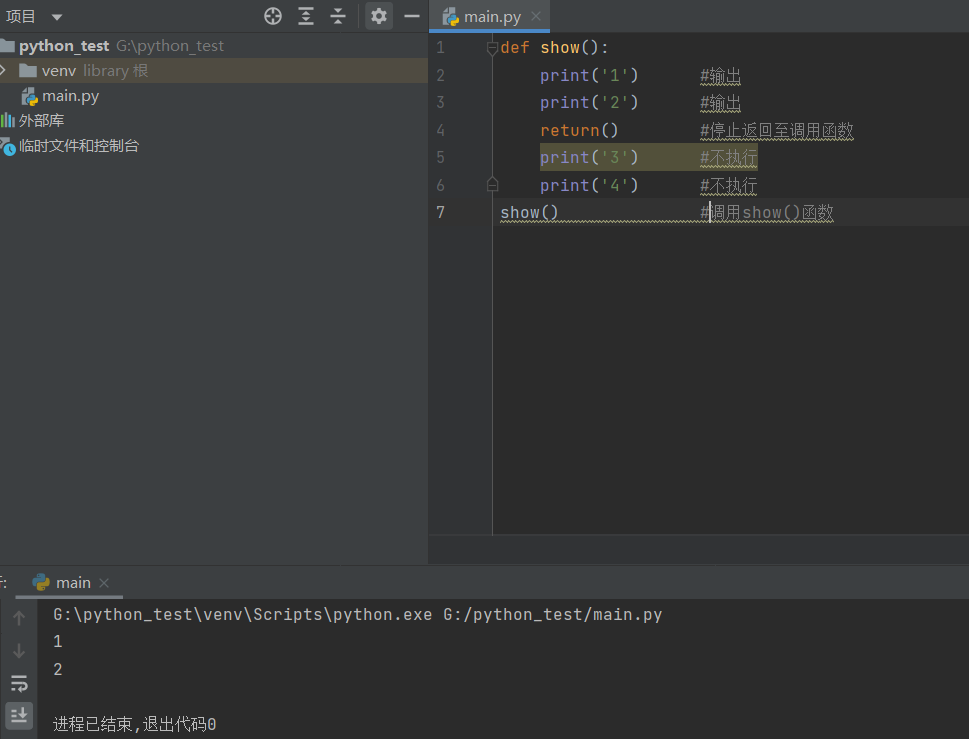`return`默认为空`none`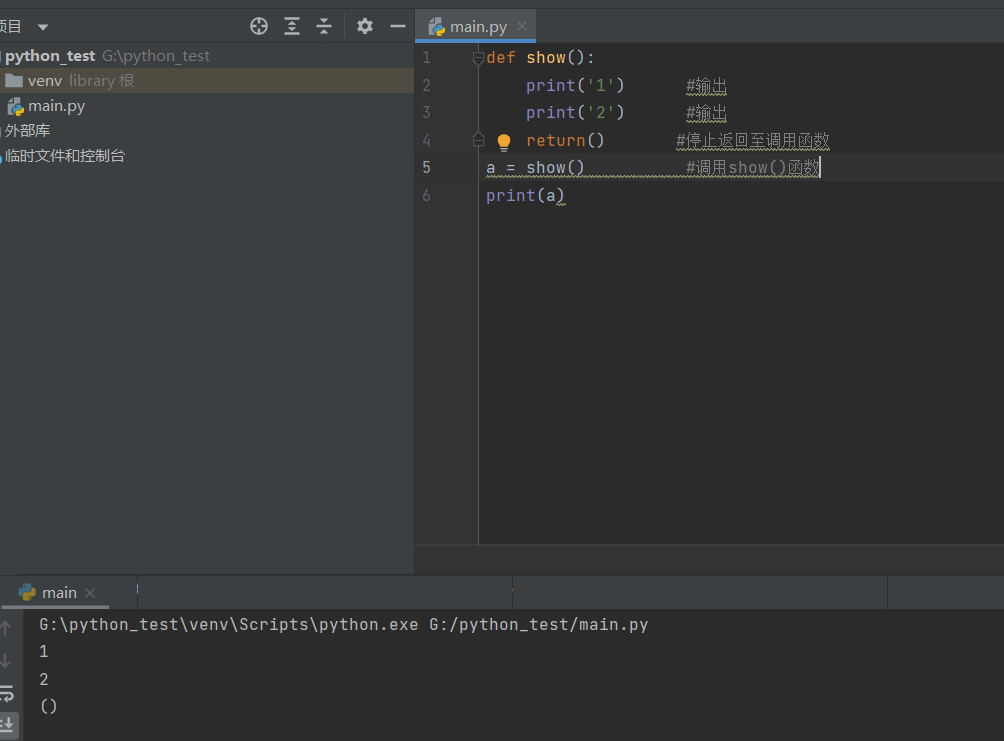``````return		  返回None

return 值 	  有打印函数 将值打印出来 且不输出None``````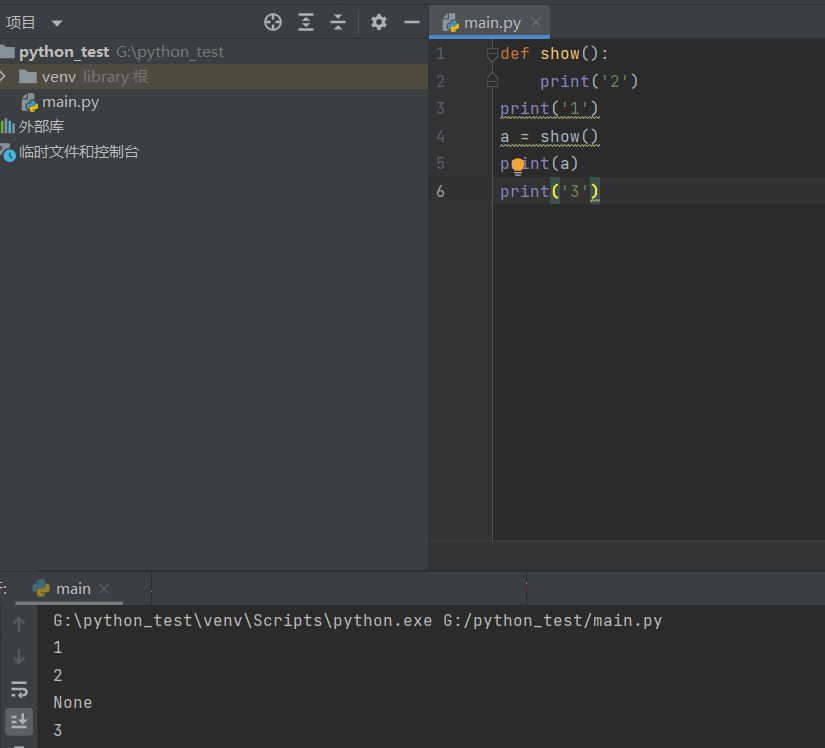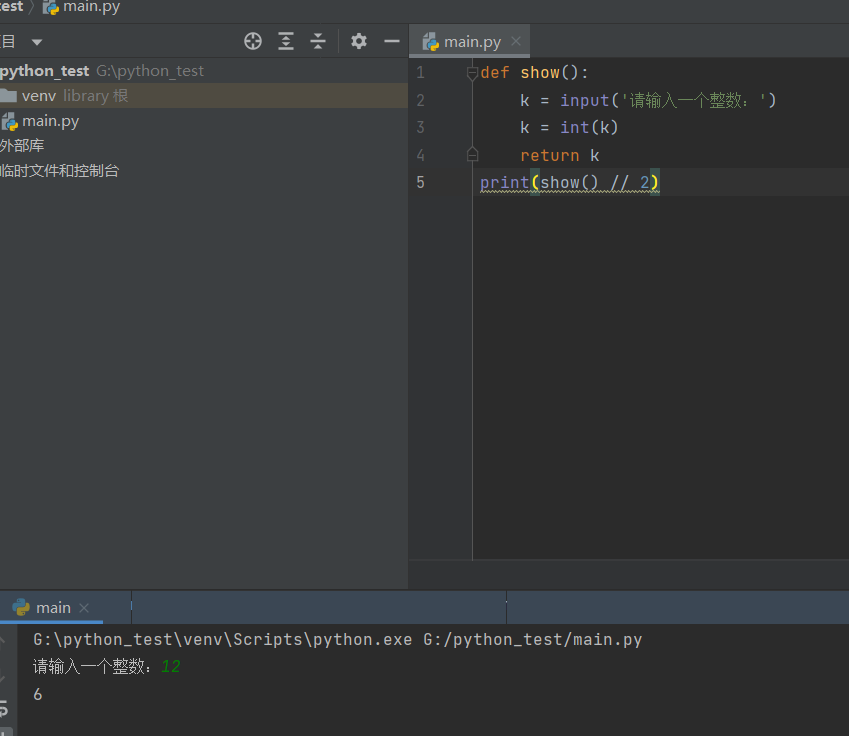## 03x13 day4

### 函数联系-传参和输入输出数据的区别

``````'''

'''
def show(a , b):
return a + b
print(show(1 , 2))

s= show( 1, 2)
print(s)``````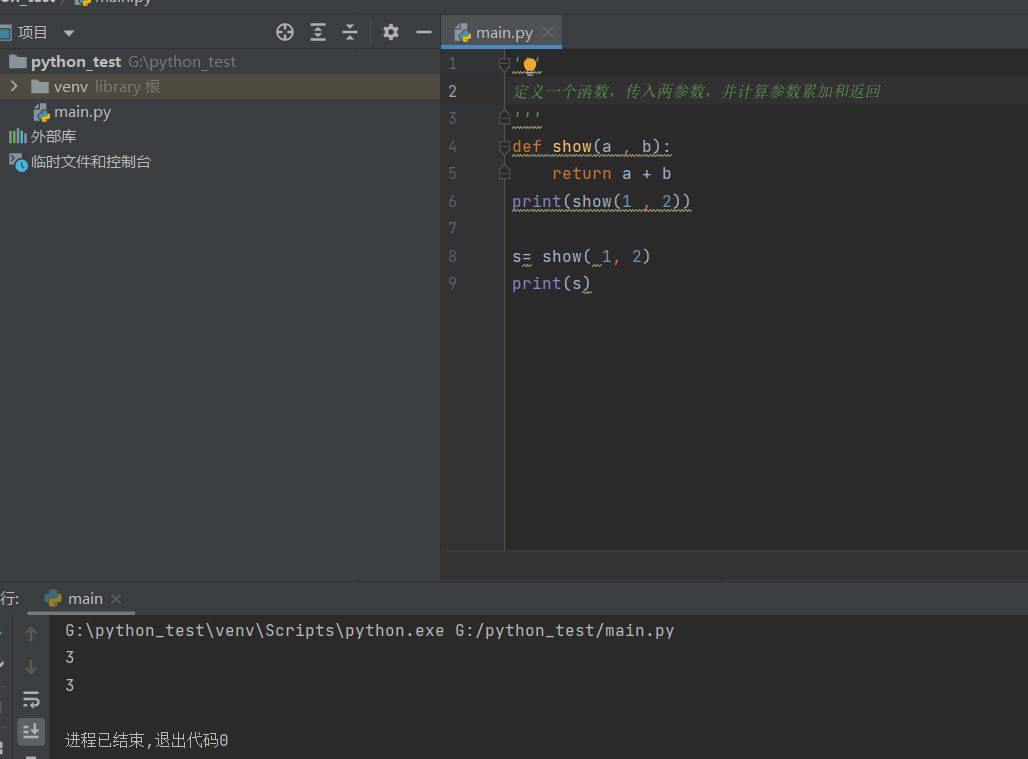### 8.函数包含多个return语句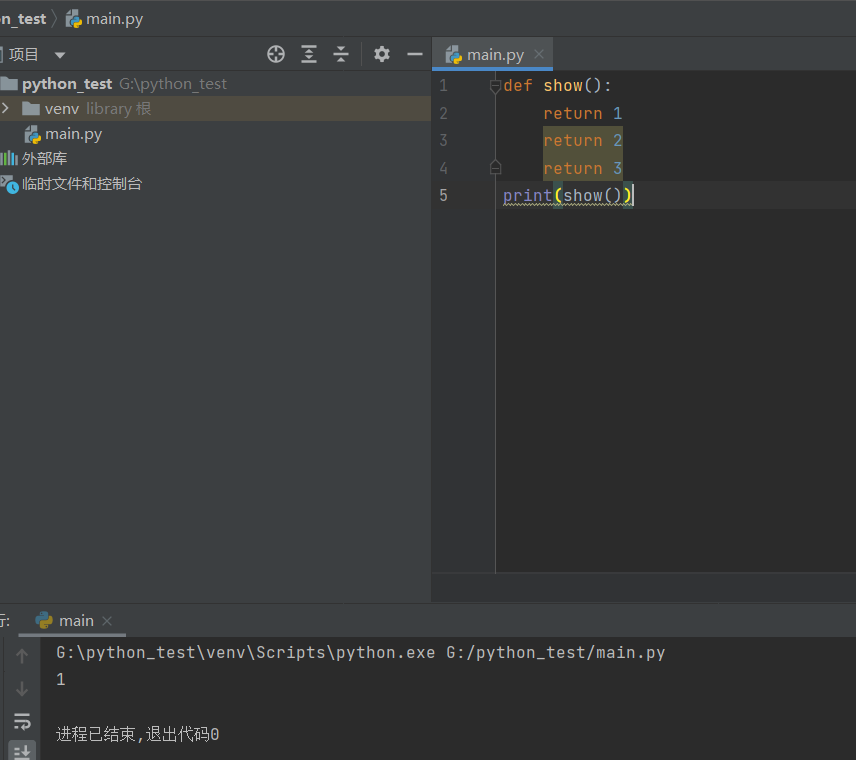### 判断语句和循环语句

1.比较（即关系，条件）运算符

python中的比较晕旋覆如下表

== 检查两个操作数的值是否相等，如果是则条件变为真 如a=3,b=3,则(a == b)为true
!= 检查两个操作数的值是否相等，如果只不相等，则条件变为真。 如a=1,b=3,则(a != b)为true
> 检查左操作数的值是否大于右操作数的值，如果是，则条件成立 如a=7,b=3,则(a > b)为true
< 检查左操作系统的值是否小于右操作数的值，如果是，则条件成立 如a=7,b=3,则(a < b)为true
>= 检查左操作系统的值是否大于或等于右操作数的值，如果是，则条件成立。 如a=3,b=3,则(a >= b)为true
<= 检查左操作数的值是否小于或等于有操作数的值，如果是，则条件成立。 如a=3,b=3,则(a <= b)为true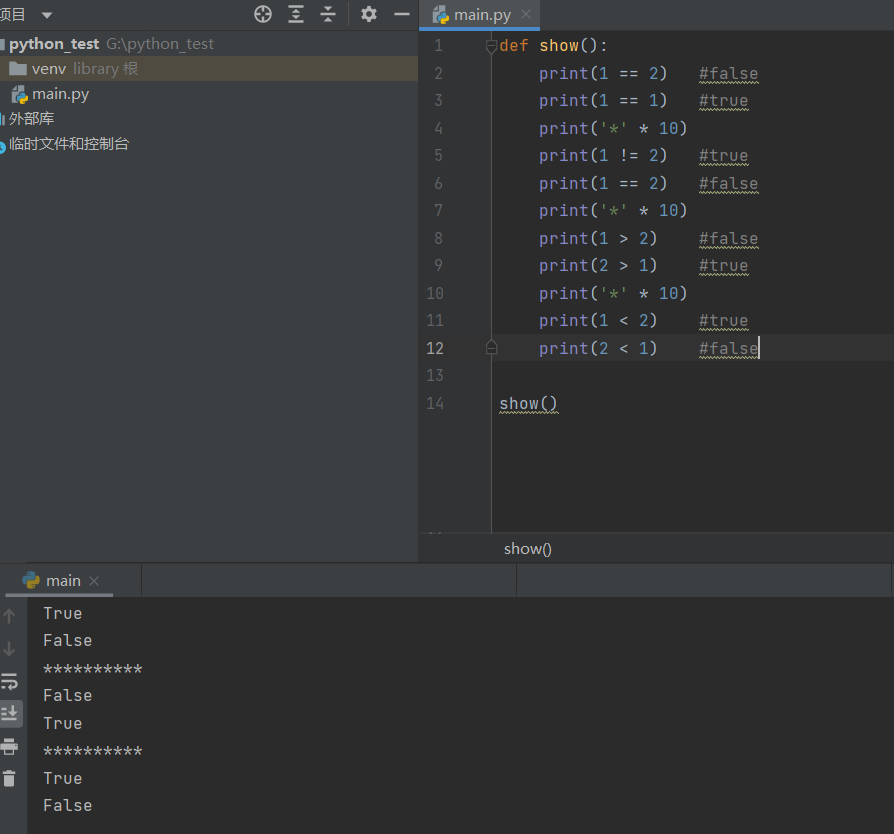2.逻辑运算符

and x and y 布尔‘与’：如果x 为false, x and y返回false,否则它返回y的值 true and false,返回false
or x or y 布尔‘或“：如果x是 true,它返回true,否则它返回y的值 false or true,返回true
not bot x 布尔”非“：如果x为true,返回false。如果x为false,它返回true not true返回false,not false返回true

### and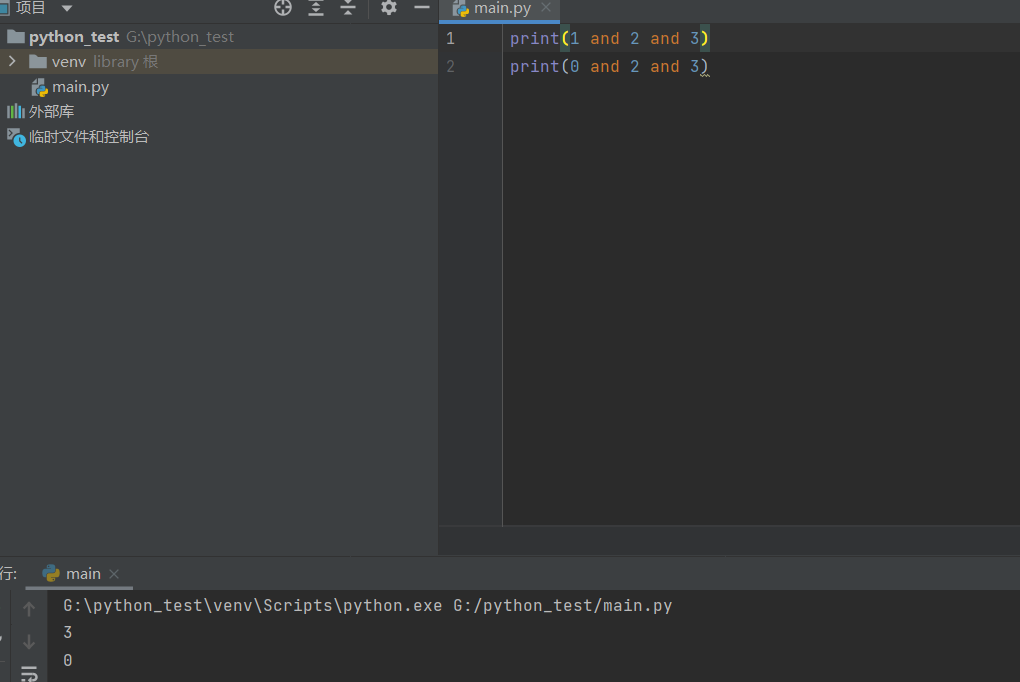### or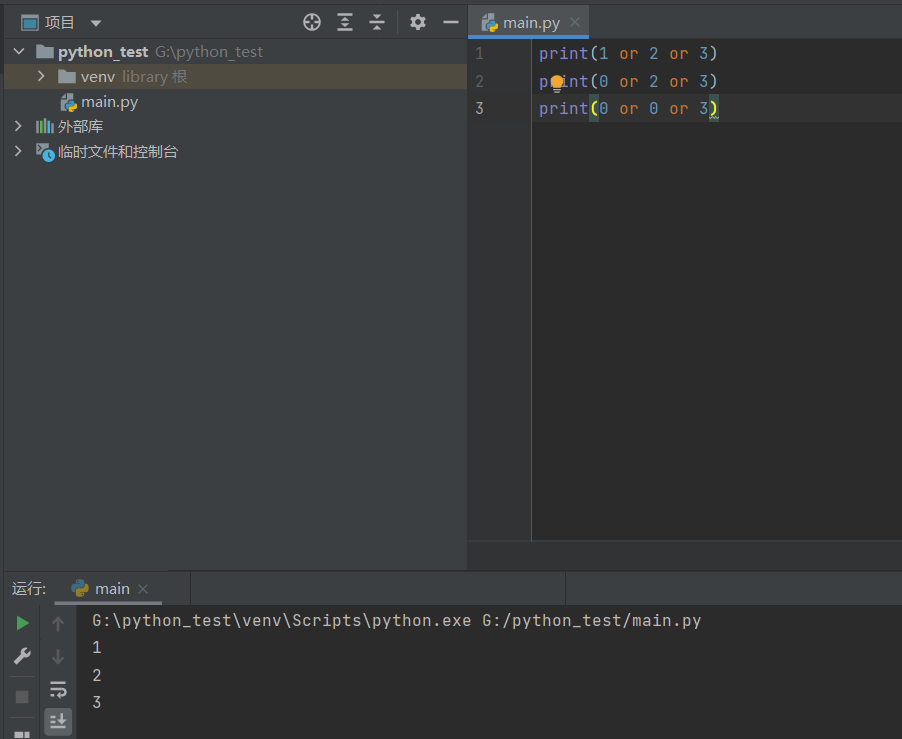### if语句的格式和作用

``````if条件
满足条件执行的代码``````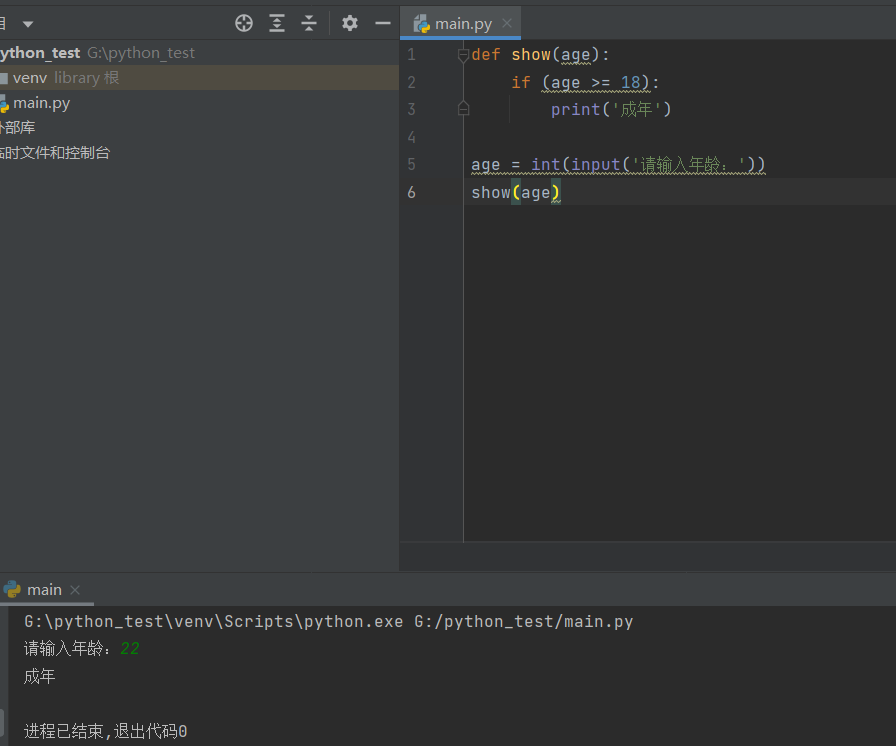if也可不加括号

### if-else格式和作用

``````if条件:
满足条件的代码
else:
不满足条件的代码``````

if判断条件，如果条件满足，执行if后面的代码，如果条件不满足，执行else后面的代码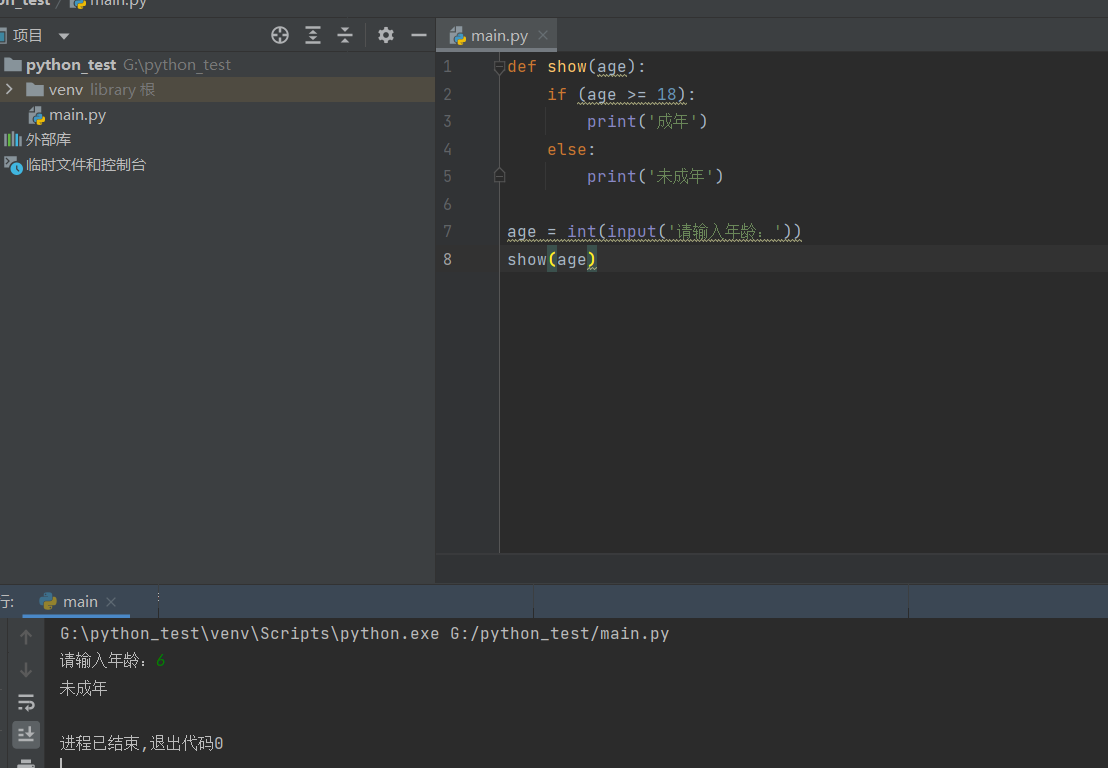``````def show(n):
if n % 2 == 0:
print(f' {n} 是偶数')
else:
print(f'{n}是基数')

m = int(input('请输入一位数'))
show(m)``````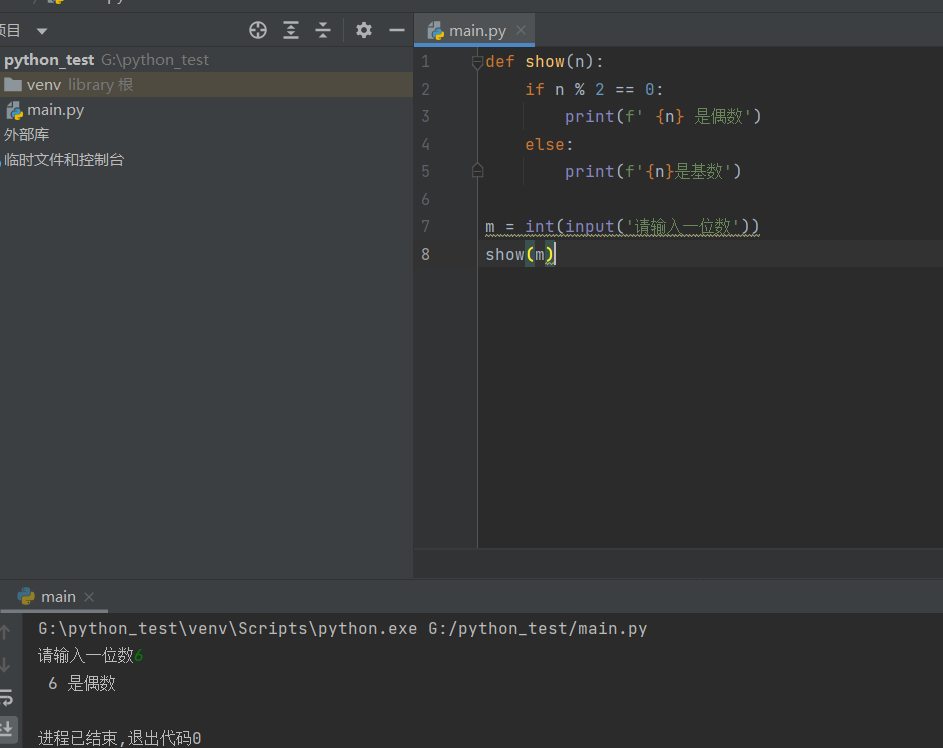``````def show(n):
if n % 2 == 0:
print('%d是偶数' %n)
else:
print(f'{n}是基数')

m = int(input('请输入一位数'))
show(m)``````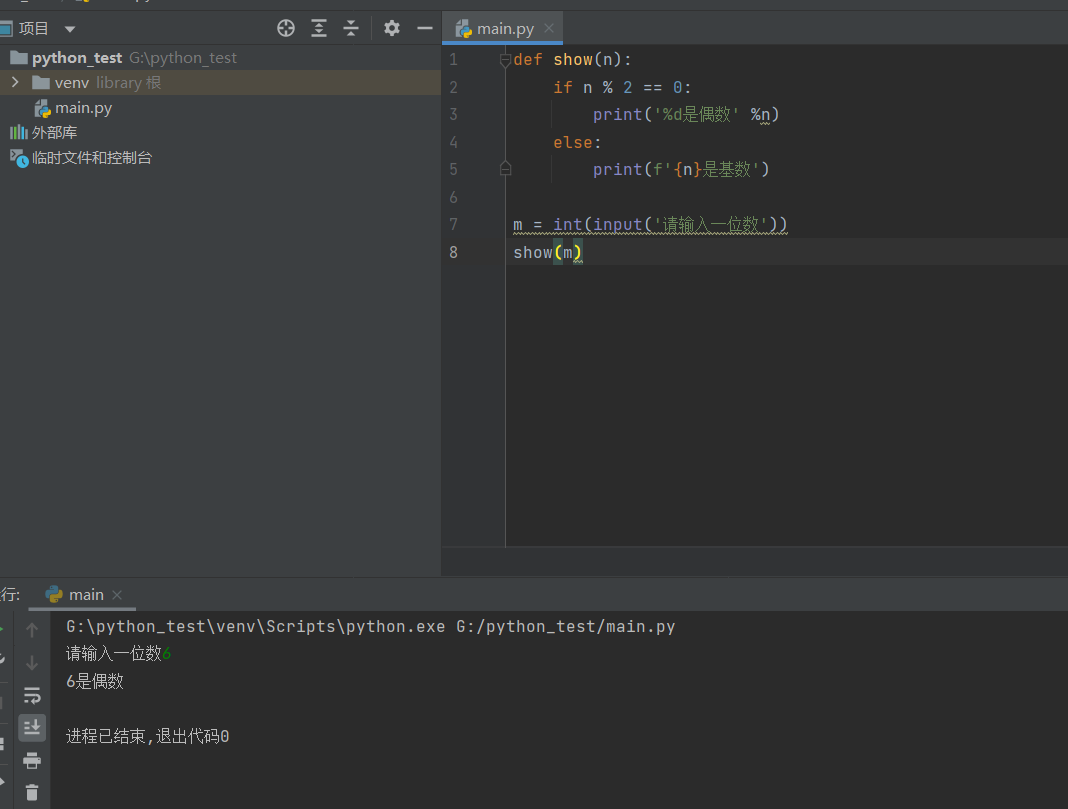### if elif else格式

``````if 条件1:
满足条件1执行的代码
elif 条件2:
满足条件2执行的代码
else:
不满足条件执行的代码``````
``````def show(day):
if day == '1' or day == '一':
print('星期一')
elif day == '2' or day == '二':
print('星期二')
elif day == '3' or day == '三':
print('星期三')
elif day == '4' or day == '四':
print('星期四')
elif day == '5' or day == '五':
print('星期五')
elif day == '6' or day == '六' :
print('星期六')
elif day == '7' or day == '七':
print('星期天')
else:
print('错误')

d = input('请输入：')
show(d)``````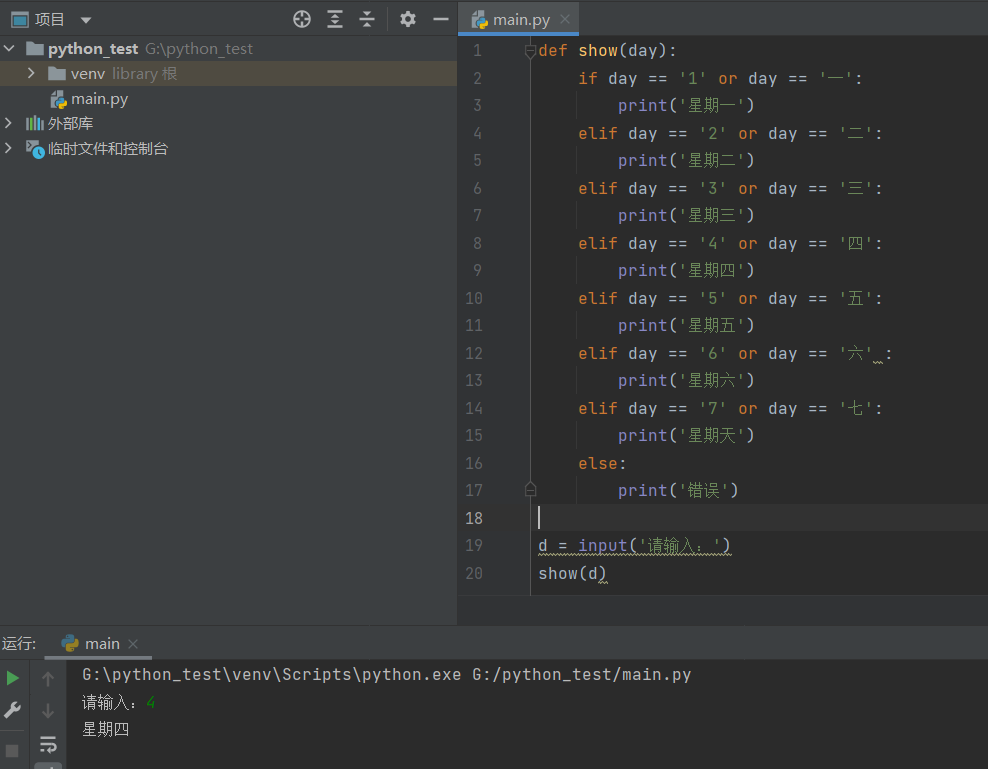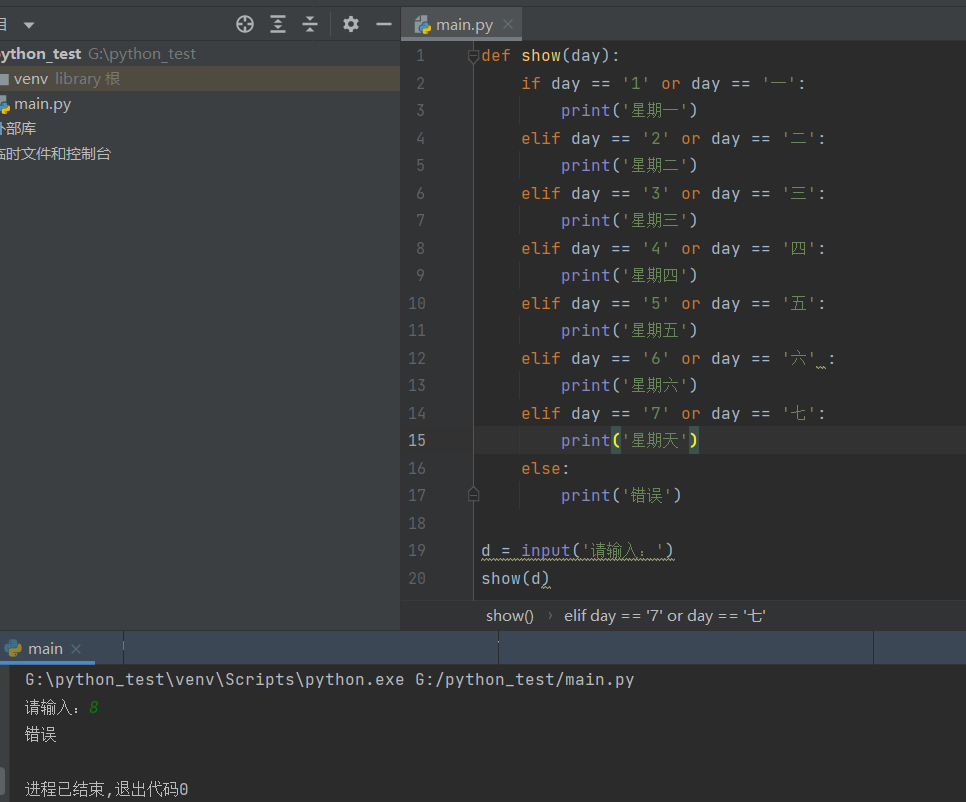### if语句的嵌套作用

``````if 条件:
if 条件:
if 条件:``````
``````def show(score):
if score >= 90:
print(f' 得分{score }, 级别优')
elif score >= 80:
print('得分 %d 级别良' %score)
elif score >= 70:
print(f'得分 {score}, 级别中')
elif score >= 60:
print(f'得分 {score}, 级别查')
else:
print(f'得分 {socre}, 不及格')

s = int(input('请输入成绩：'))
show(s)``````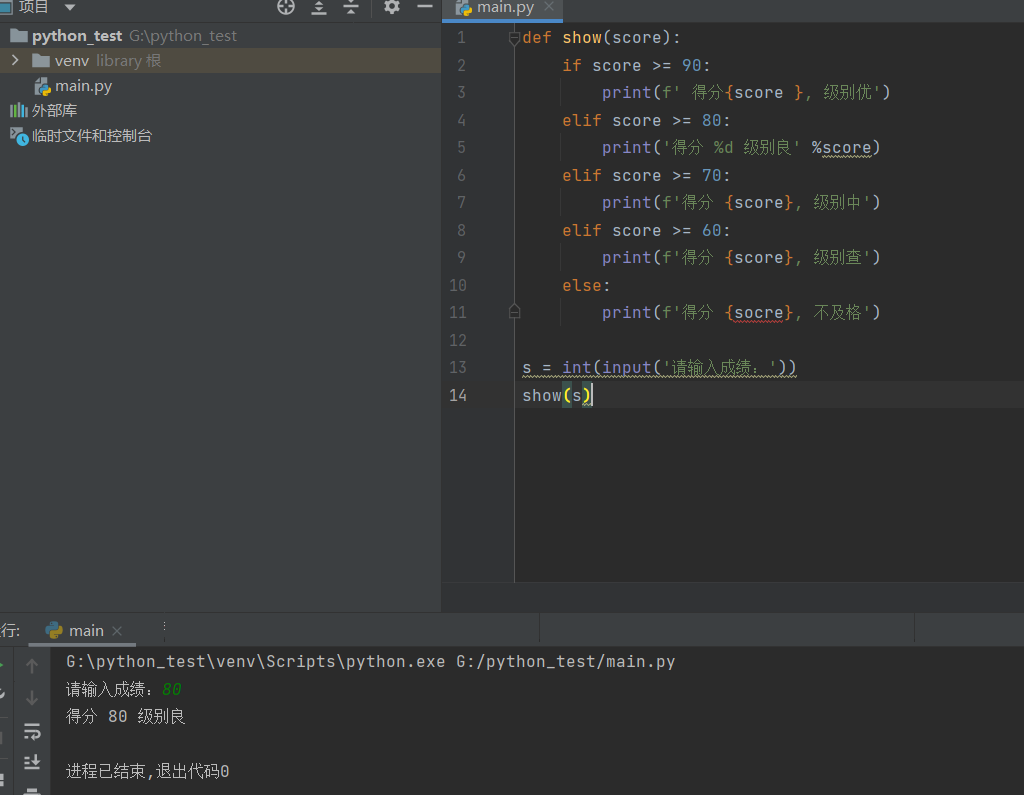``````def show(score):
if score >= 0 and score <= 100:     #成绩有效判断
if score >= 90:
print(f' 得分{score }, 级别优')
elif score >= 80:
print('得分 %d 级别良' %score)
elif score >= 70:
print(f'得分 {score}, 级别中')
elif score >= 60:
print(f'得分 {score}, 级别查')
else:
print(f'得分 {socre}, 不及格')
else:
print(f'成绩{score}是无效的')

s = int(input('请输入成绩：'))
show(s)``````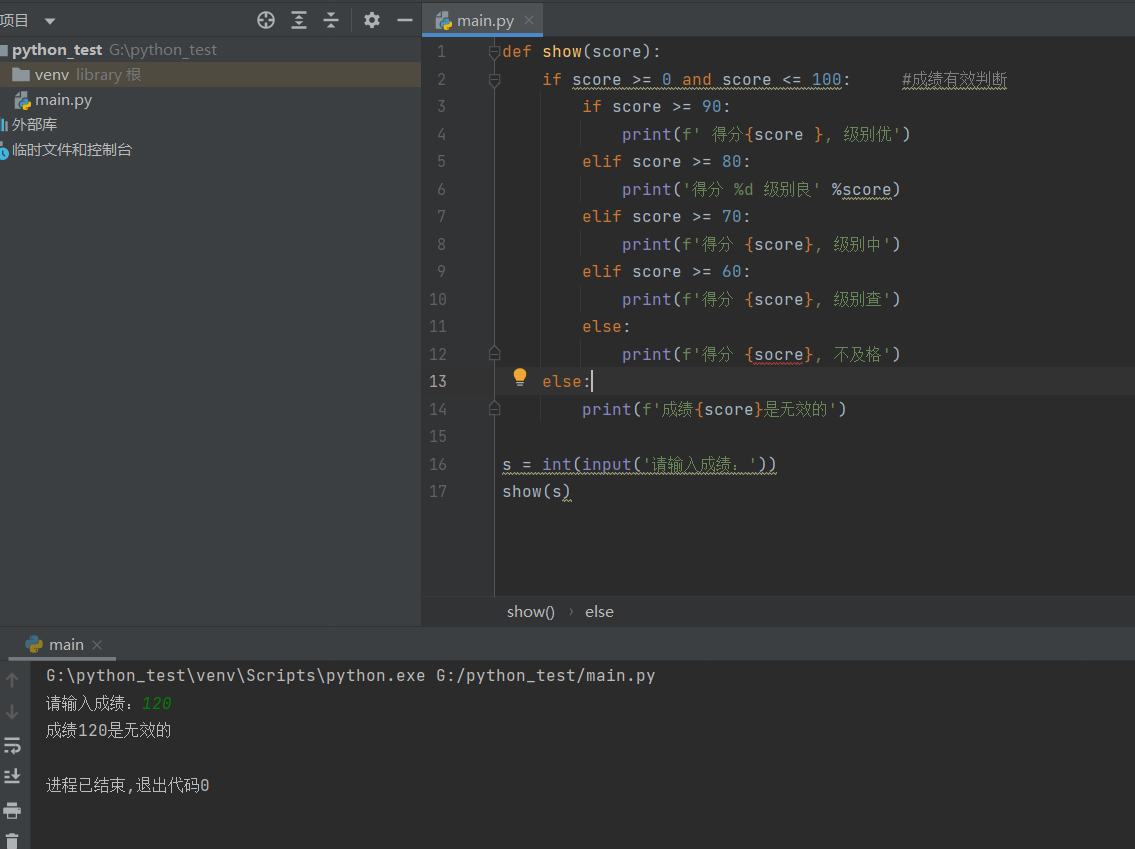### 随机数Random

1.导入 `random` 模块

1. `random.randint(n1,n2)`

通过这个函数得到一个`[n1,n2]`的区间 比如`[1,9]`会得到1-9随机数

``````import random   #导入模块

n = random.randint(1,9)     #使用随机数模块获取一个随机数
print(n)``````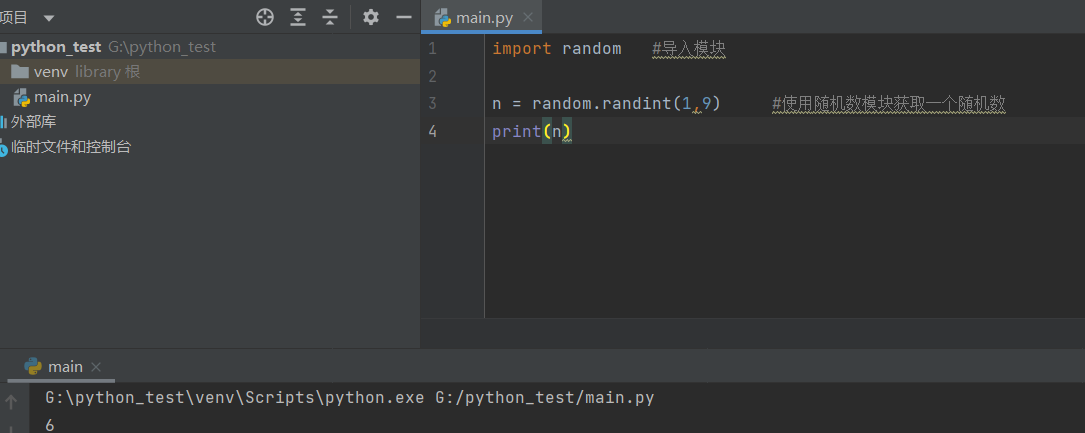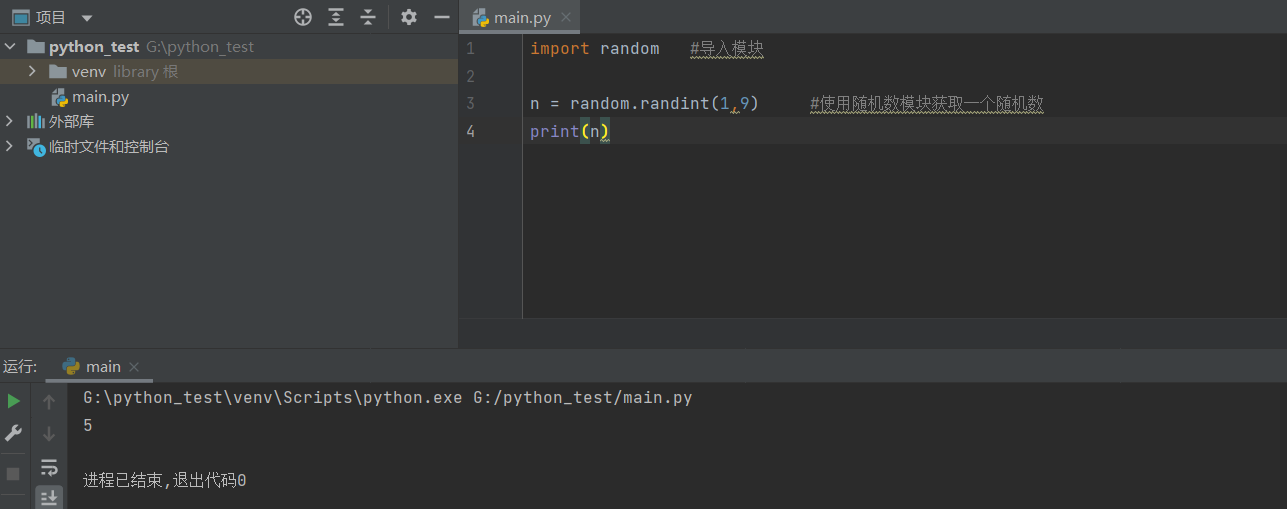## 03x14 day5

### break

1.break 只能用在循环里

2.break 的作用是用来结束循环，不管循环多少次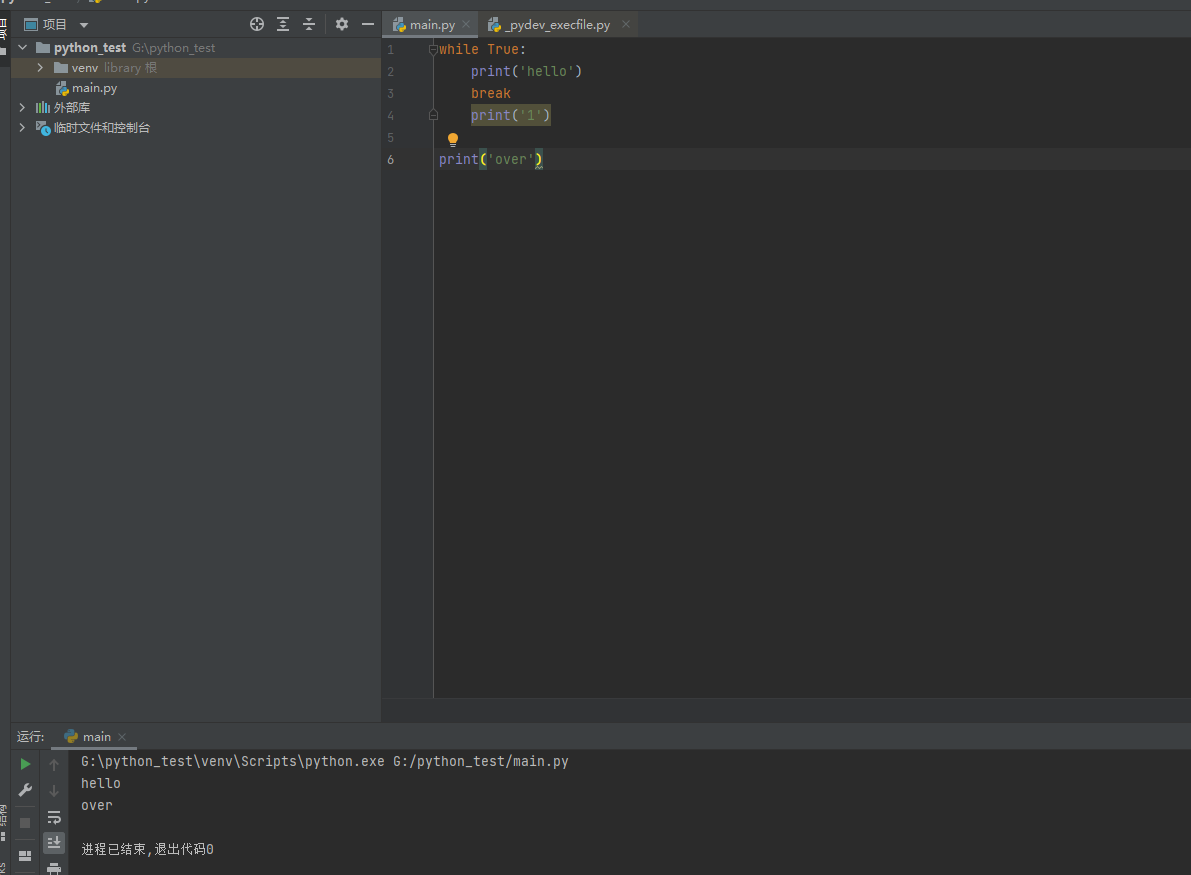### continue

1. continue 也只能用在循环里
2. continue得作用是用来结束本次循环，不管循环体中还有多少代码没执行 ，进入下一次循环
``````while True:
print('hello')
continue
print('world')``````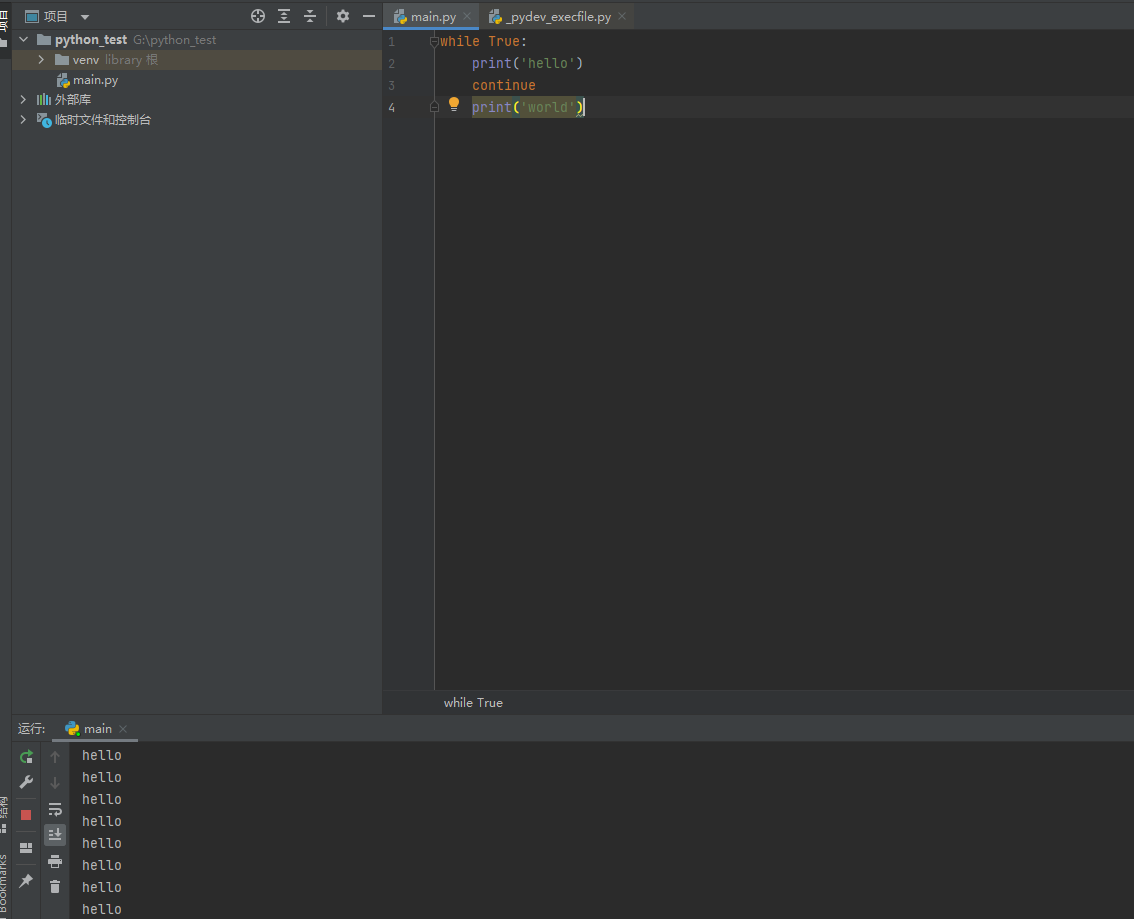## 练习：

#### 1.猜拳游戏

​ 条件

​ a.两个角色 玩家-电脑

​ b.动作：石头 0 ，剪刀 1 ，布 2

​ c.我的出拳：由输入完成

​ d.电脑的出拳：随机数完成

​ e. 比较出拳

​ f. 相等 - 平局

​ g. 玩家赢：p0:r1 p1:r2 p2:r0

​ h.剩下的情况就是电脑赢

``````'''
a. 两个角色  玩家 player  - 电脑 robot
b. 动作: 石头 0 , 剪刀 1,  布 2
c. 我的出拳: 由输入完成
d. 电脑的出拳: 随机数完成
e. 比较出拳
f. 相等 - 平局
g. 玩家赢: p0:r1  p1:r2  p2:r0
h. 剩下的情况就是电脑赢

'''

# 导入随机数模块
import random

# 定义一个函数
def game():
# 定义一个玩家变量,从键盘输入值
player = int(input('请输入一个状态(石头 0 , 剪刀 1,  布 2):'))

# 定义一个电脑变量,使用随机数获取状态
robot = random.randint(0, 2)

# 比较状态
# 先状态平局
if player == robot:
print('平局')
# 再判断玩家赢的状态
elif ((player == 0) and (robot == 1)) or ((player == 1) and (robot == 2)) or ((player == 2) and (robot == 0)):
print('玩家赢')
# 剩下就是电脑赢的状态
else:
print('你输出,电脑胜出')

# 函数调用,开始游戏
game()
``````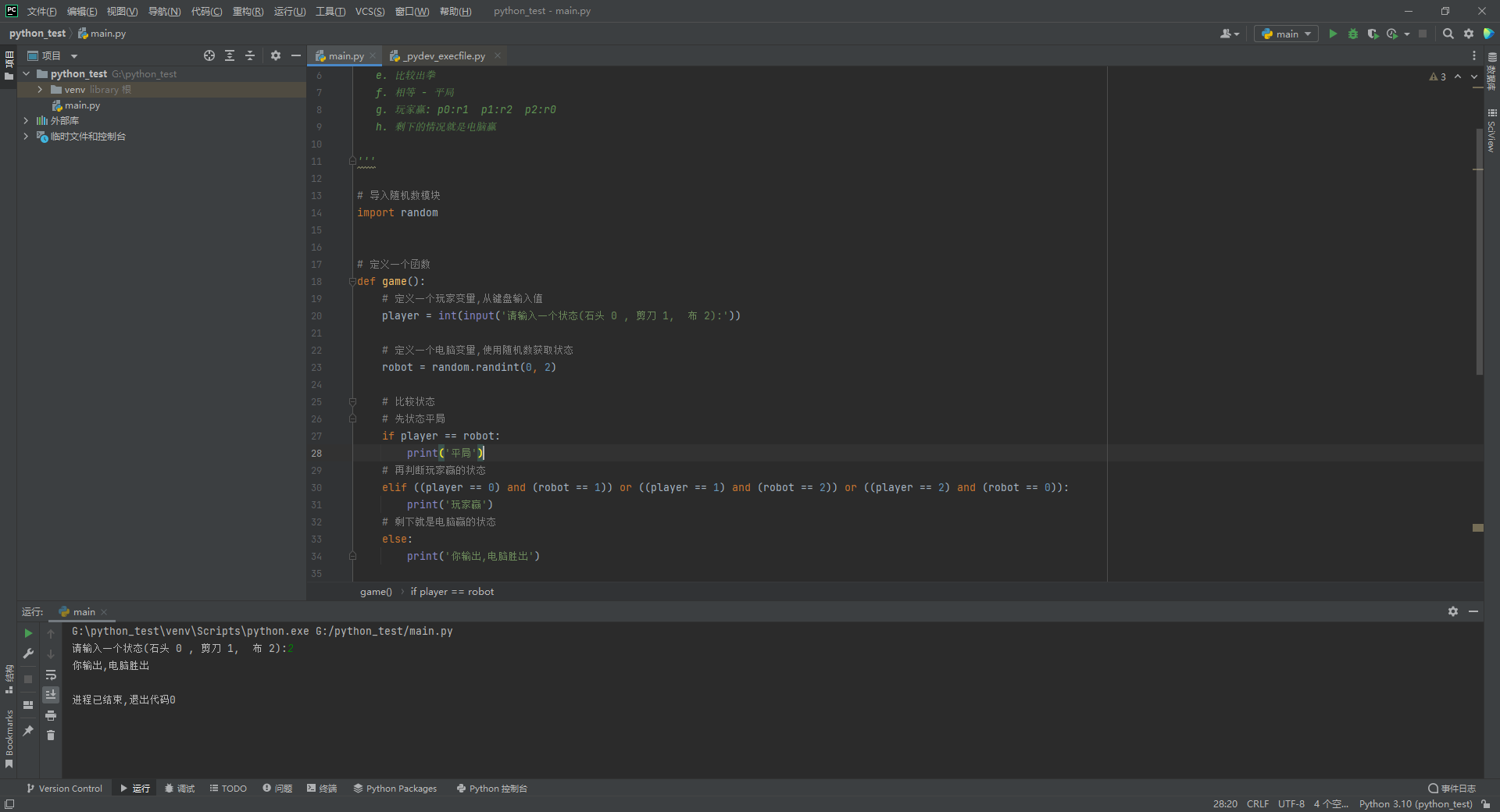#### 2.输出矩形

``````'''

'''

#定义一个矩形
def show():
i =0
while i < 5:
j = 0
while j < 5:
#在print函数中，使用 end= 形式来给print函数设置一个结束符号，默认的结束符是\n换行
print(' * ', end=' ')
j += 1
print() #起到默认换行作用
i += 1

show()``````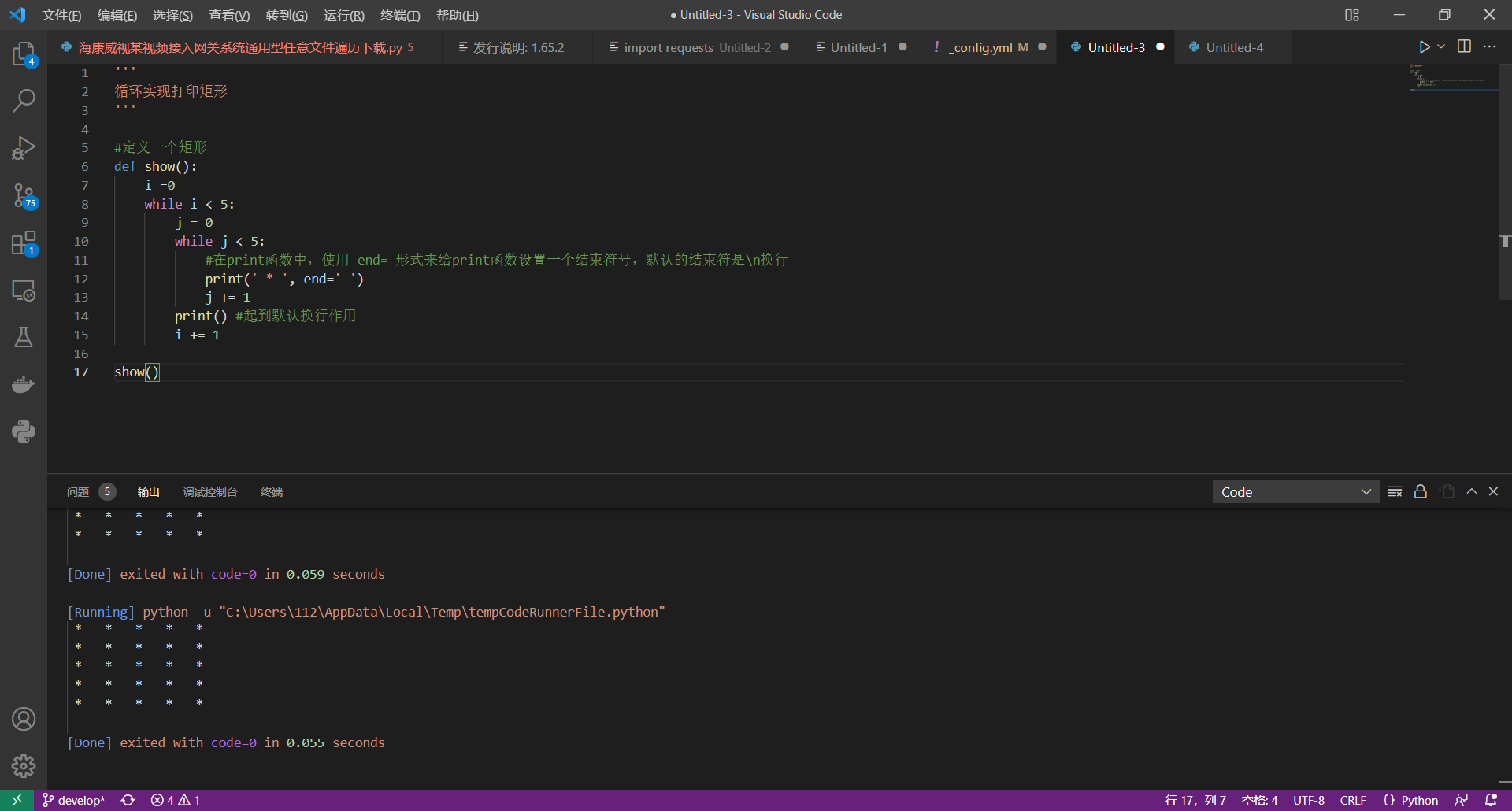## 03x15 day6

### 三目运算符

c语言：`条件？表达式1：表达式2`

python: `表达式1 if 条件 else 表达式2`

``````def show():
n = int(input('number:'))

s = '偶数' if n % 2 == 0 else '奇数'
print(s)

show()``````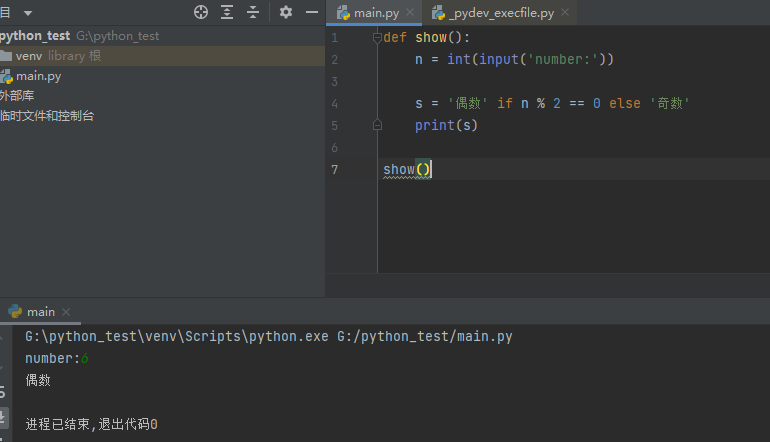## 03x16

### 循环语句while

​ 1.顺序结构—–从上至下。顺序执行

​ 2.分支结构—–根据条件是否成立，选择不同的代码执行

​ 3.循环结构—–一段代码会重复执行多次

​ 1.循环初始值

​ 2.循环条件

​ 3.循环体代码

​ 4.让循环变量趋近于结束条件的代码

​ 1 > 2 > 3 > 4 > 2 > 3 > 4 > 2 > 3 > 4 > ….2(不满足条件，退出循环)

while格式

``````while条件：

​		循环体代码``````
``````'''

'''
def show():
#定义一个循环变量并有初值
i = 1

#判断条件
while i <= 100:
print(i)
i += 1

show()``````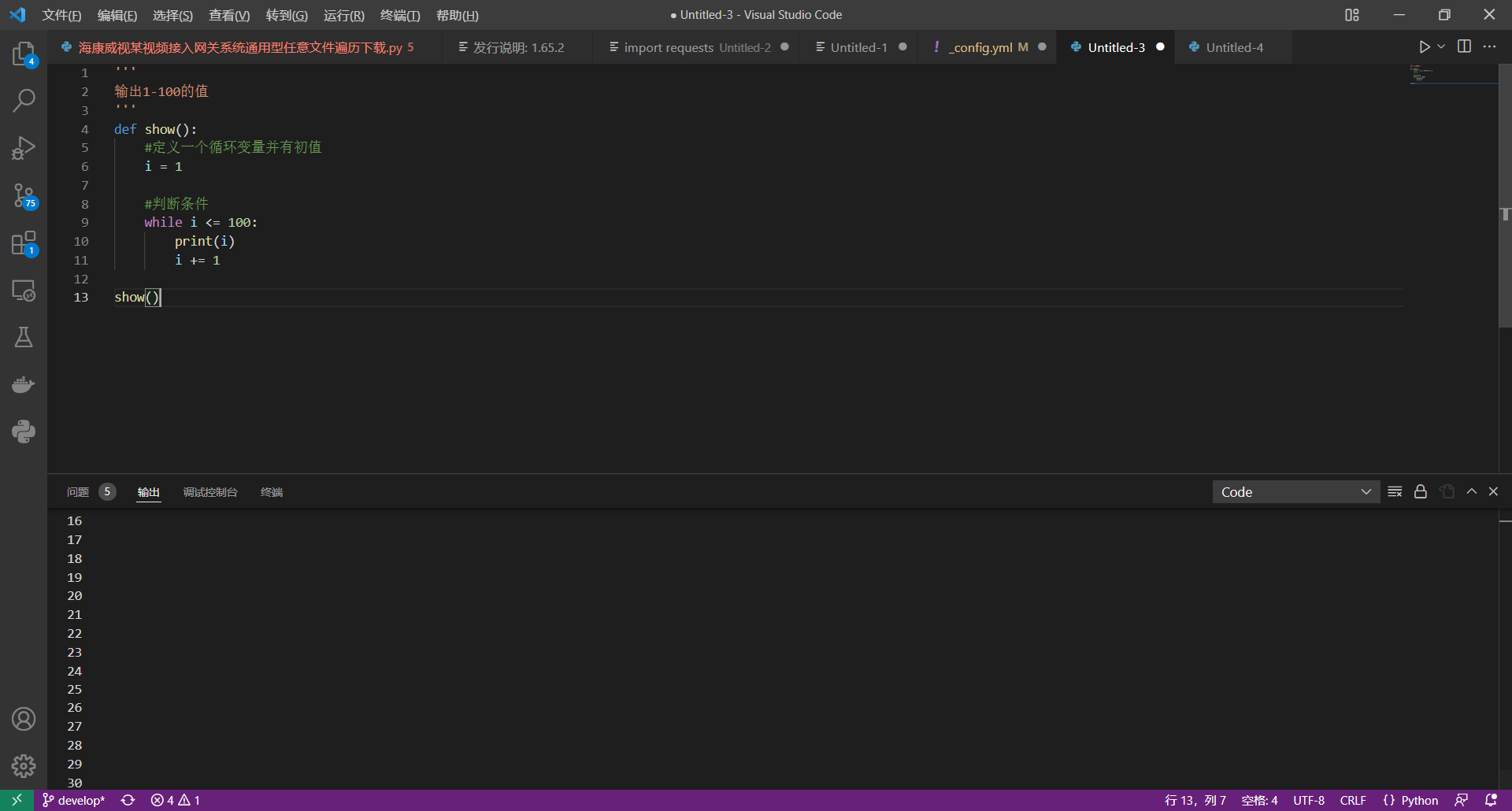### while循环嵌套

``````while 条件1:
while 条件2:
循环体...``````

``````'''

'''

i = 0
while i < 3:
print('i--', i)
j = 0
while j < 5:
print('j---', j)
j += 1
i += 1``````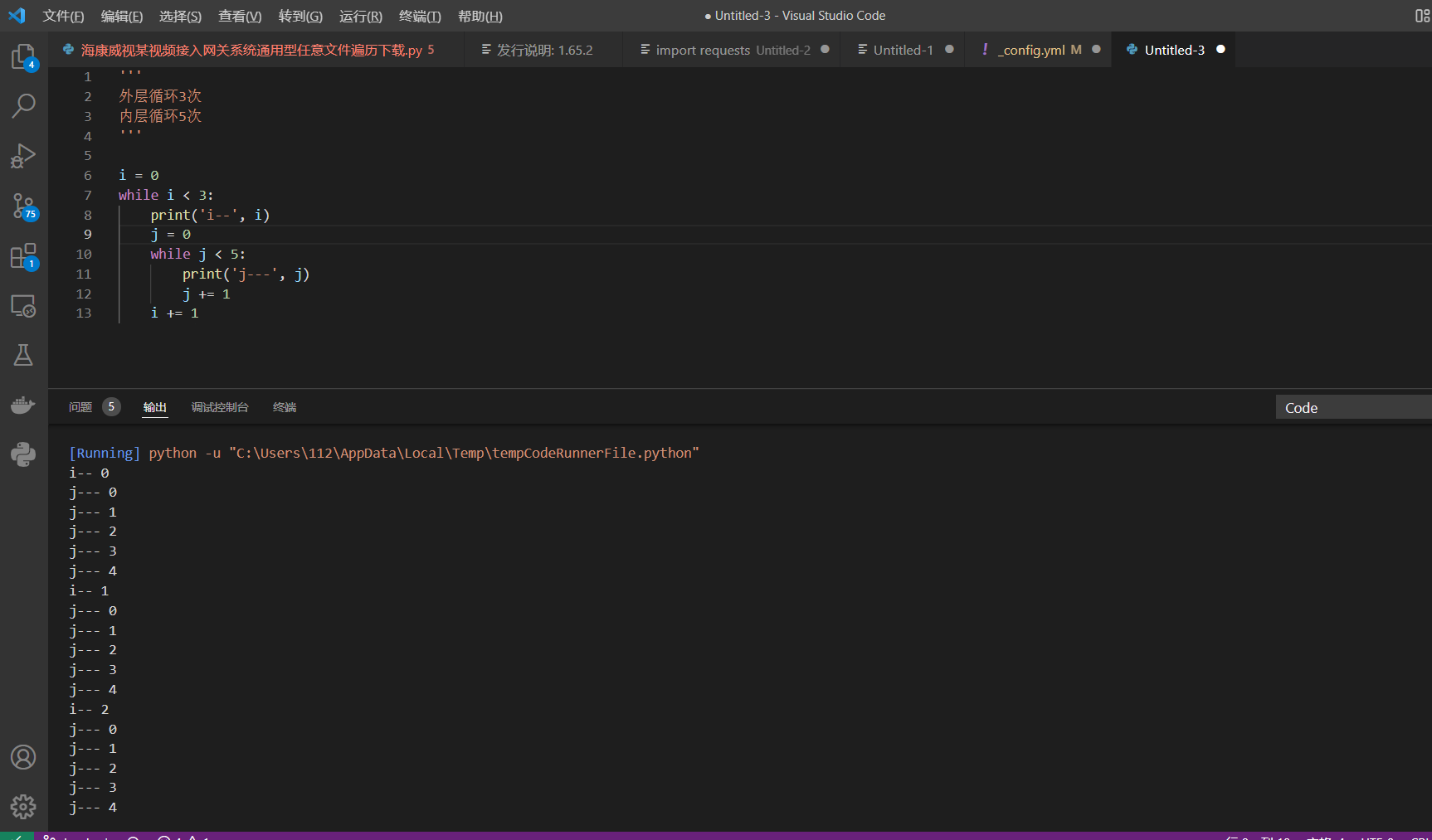### 多层循环中使用break和continue

``````'''

'''

i = 0
while i < 3:
print('i--', i)
j = 0
while j < 5:
print('j---', j)
break
j += 1
i += 1``````

`break`会跳出最近得所在得循环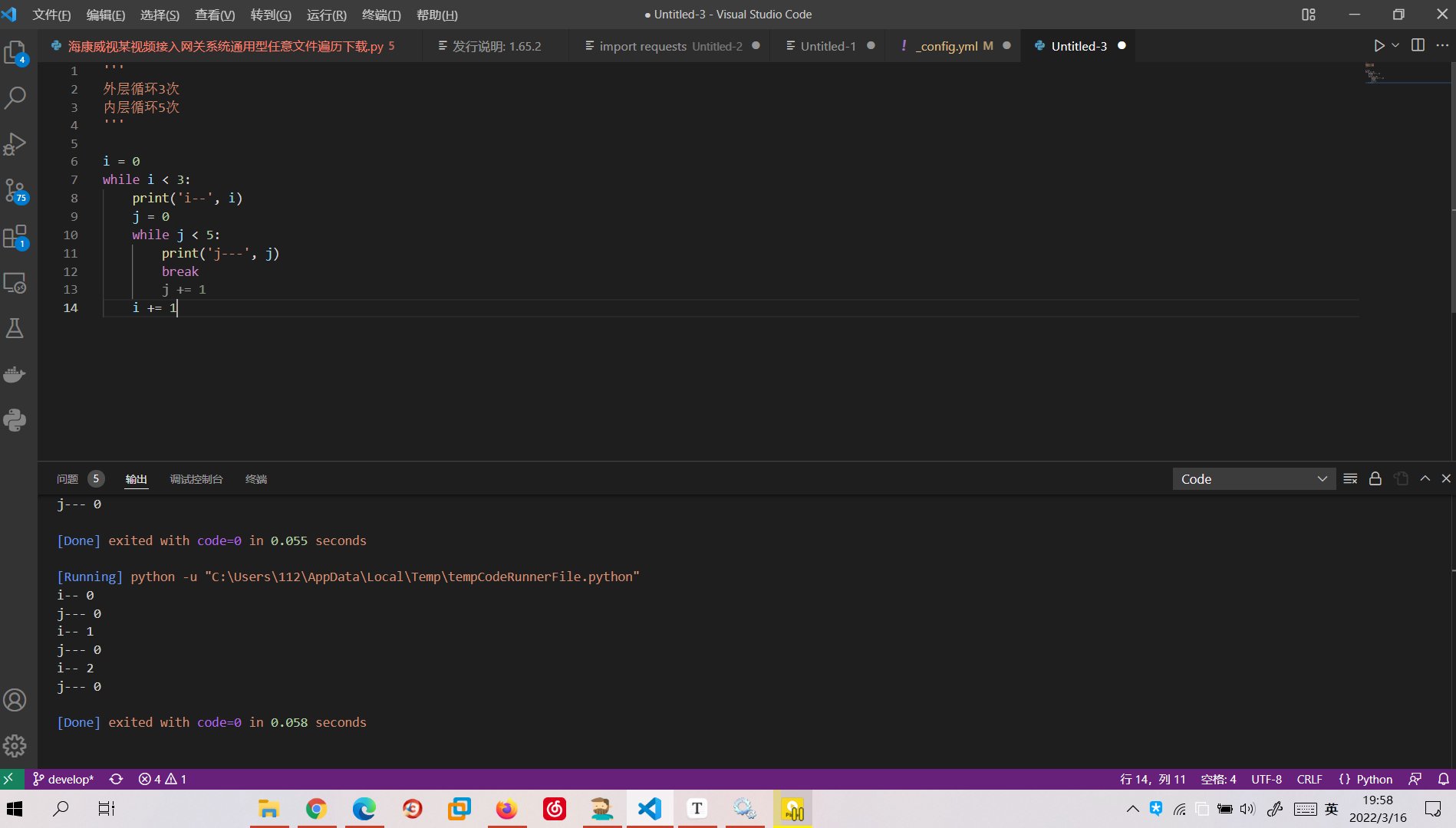`break``j += 1`前面跳出循环执行外层`i`的循环 所以j的值不会发生变化 i的条件是3所以i,y执行三次

``````def show(n):
i = 0
while i < n:
#if i % 2 == 0
#print(i)
i += 1
if i % 2 ==1:
continue
print(i)

show(10)``````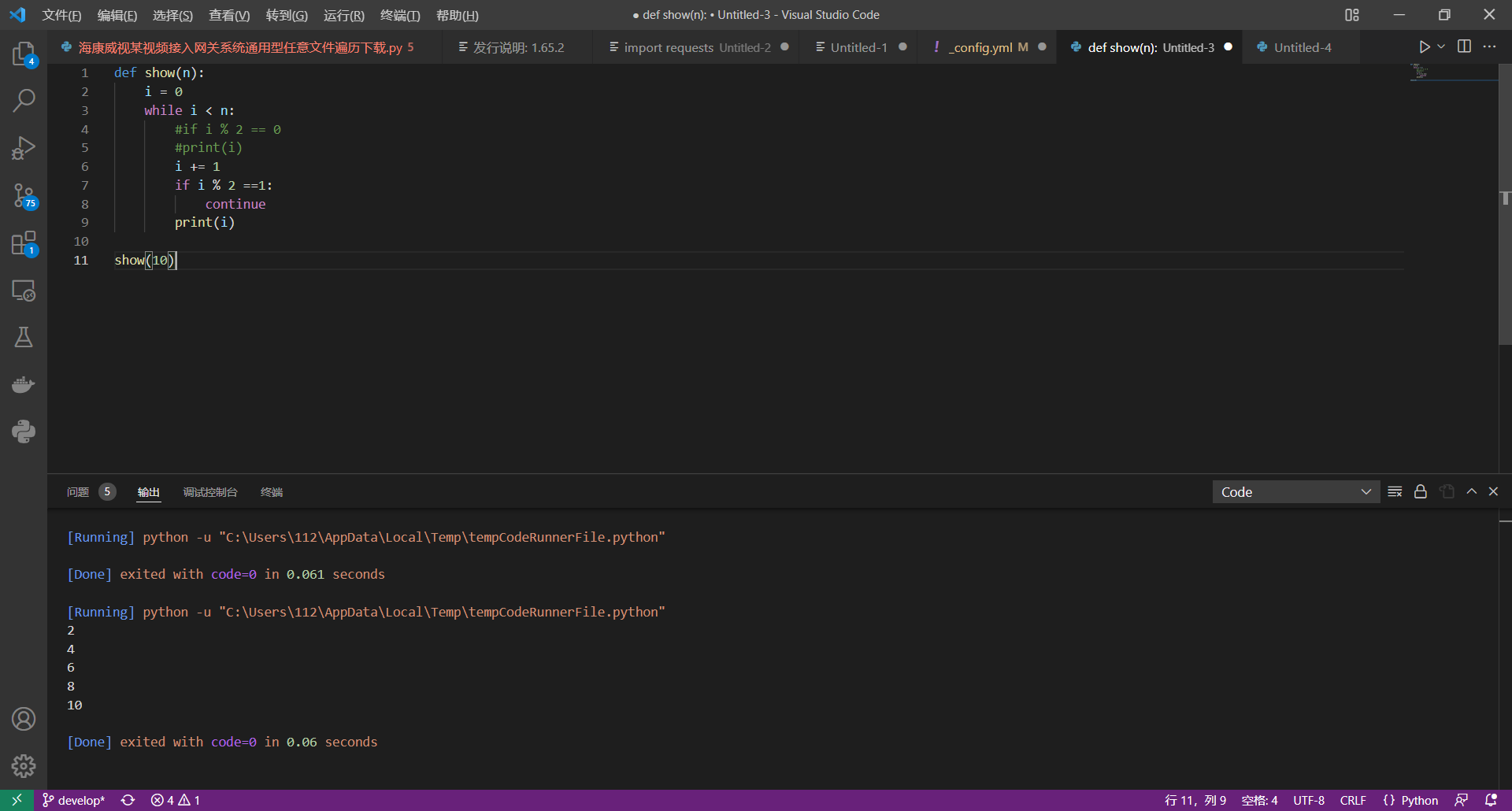目录